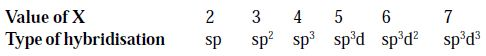# How to Predict the Type of Hybridization in a Molecule or Ion

Predict the Type of Hybridization in a Molecule or Ion

Step 1: Add the number of valence electrons of all the atoms present in the given molecule/ion.

Step 2: In case of a cation, subtract the number of electrons equal to the charge on the cation and in case of an anion, add number of electrons equal to the charge on the anion.

Step 3: (i) If the result obtained in step 2 is less than 8, divide it by 2 and find the sum of the quotient and remainder.

(ii) If the result obtained in step 2 lies between 9 and 56, divide it by 8 and find the first quotient (Q1). Divide the remainder R1 (if any) by 2 and find the second quotient (Q2). Add all the quotients and the final remainder (R2).

Let the final result obtained in (i) or (ii) be X. The type of hybridisation is decided by the value of X as follows:Example

(i) BeCl2

Total valence electrons = 2 + 7 × 2 = 16

16/8 = 2 (Q1) + Zero (R1); X = 2

so, Hybridization = sp

(ii) BF3

Total valence electrons = 3 + 7 × 3 = 24

24/8 = 3(Q1) zero(R1); X = 3

so, Hybridization = sp2

(iii) NH3

Total valence electrons = 5 + 3 = 8; X = 4

so, Hybridization = sp3